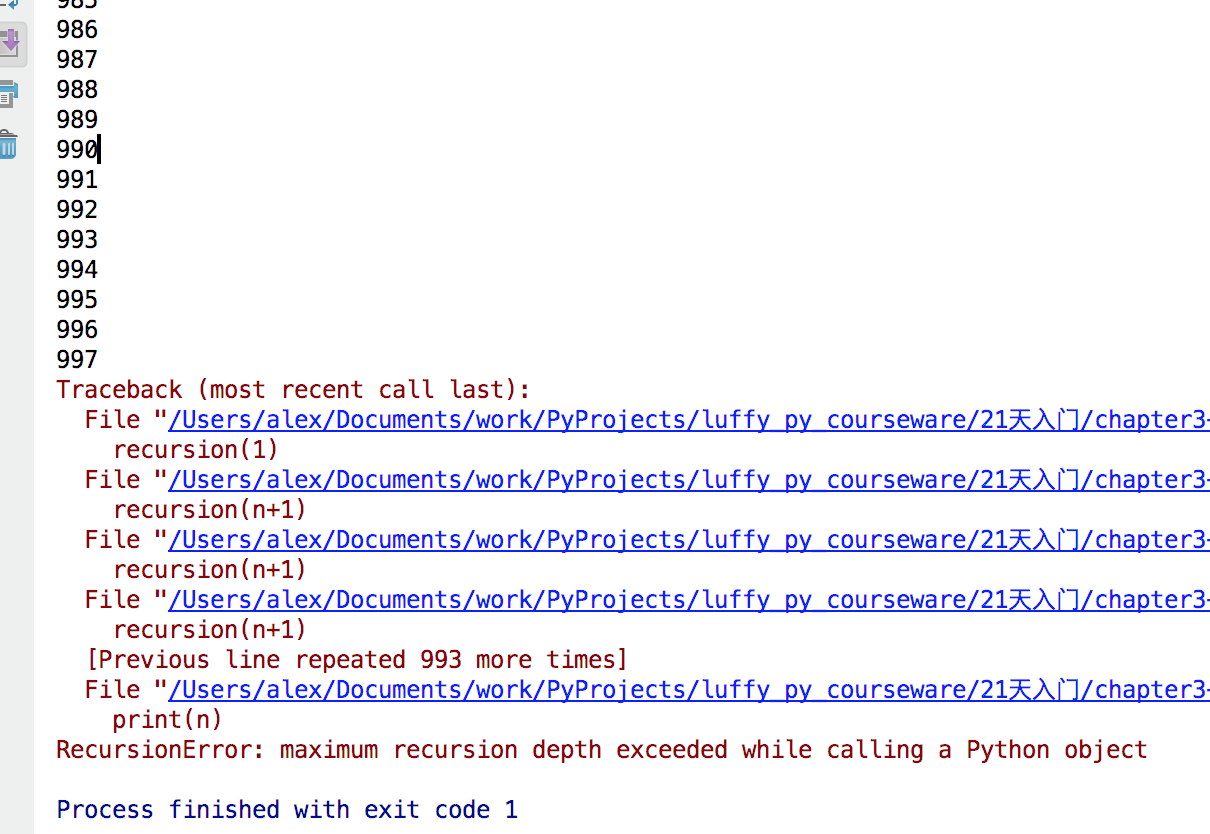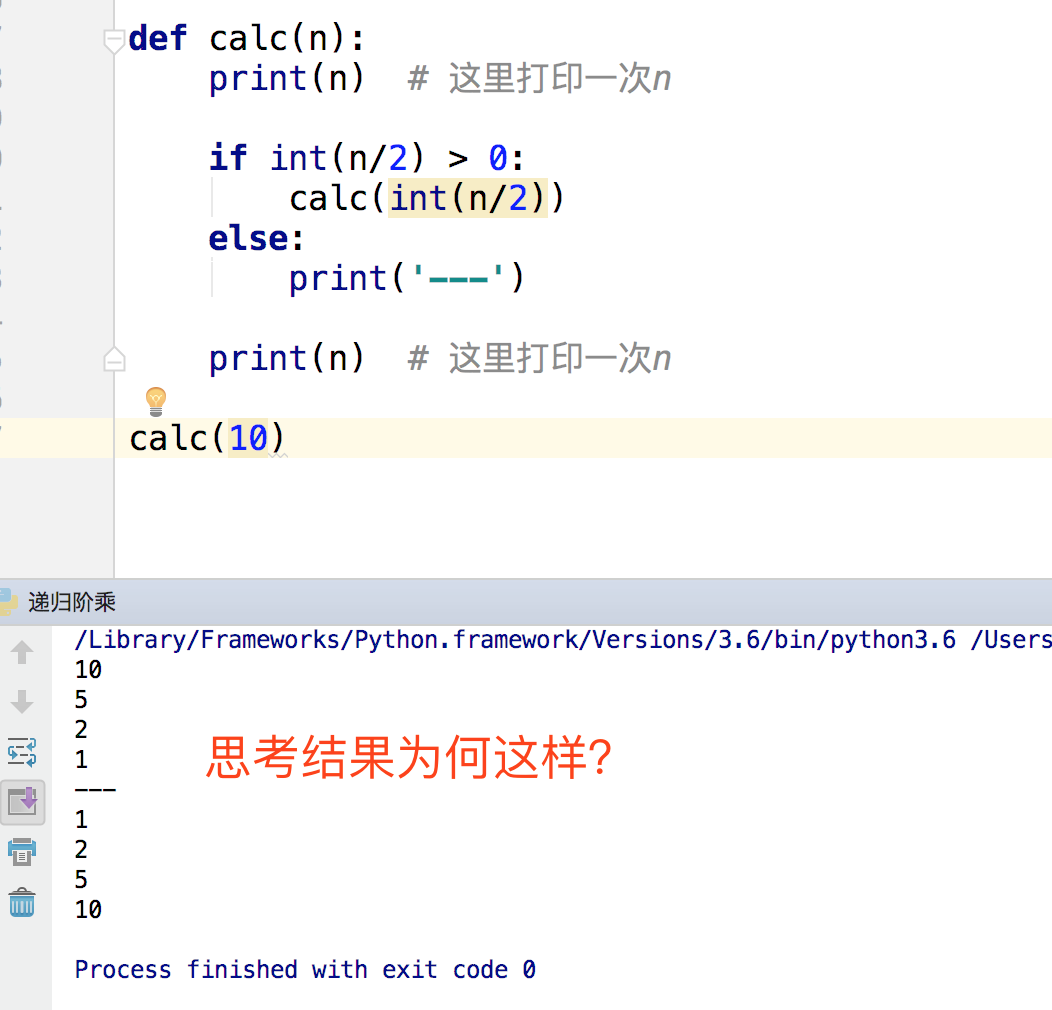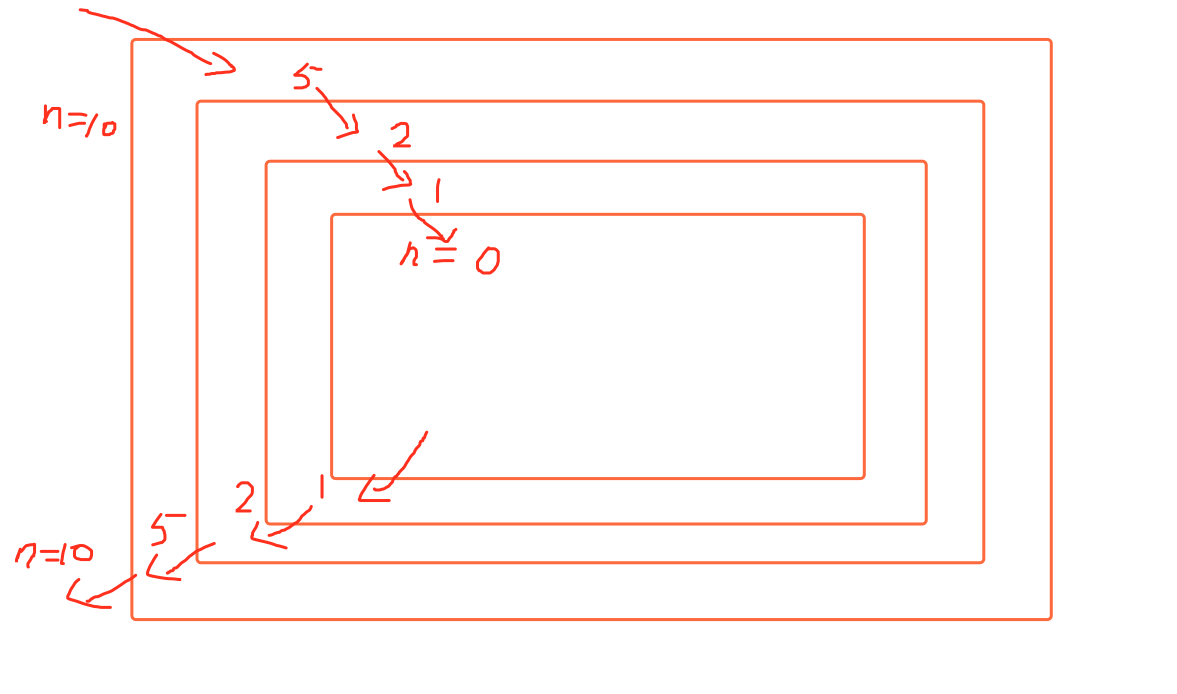# Python3-递归函数

2018/12/23 19:33

### 什么是递归？

#### 代码示例

``````def recursion(n):

print(n)
recursion(n+1)

recursion(1)　　``````### 递归的特点1. 必须有一个明确的结束条件，要不就会变成死循环了，最终撑爆系统
2. 每次进入更深一层递归时，问题规模相比上次递归都应有所减少
3. 递归执行效率不高，递归层次过多会导致栈溢出

### 递归有什么用呢？

#### 求阶乘

n!=1×2×3×……×n

n!=n×(n-1)!

``````def factorial(n):

if n == 0: #是0的时候，就运算完了
return 1
return n * factorial(n-1) # 每次递归相乘，n值都较之前小1

d = factorial(4)
print(d)``````

#### 2分查找

``````data_set = list(range(101))

def b_search(n,low,high,d):

mid = int((low+high)/2) # 找到列表中间的值
if low == high:
print("not find")
return
if d[mid] > n: # 列表中间值>n, 代数要找的数据在左边
print("go left:",low,high,d[mid])
b_search(n,low,mid,d) # 去左边找
elif d[mid] < n: # 代数要找的数据在左边
print("go right:",low,high,d[mid])
b_search(n,mid+1,high,d) # 去右边找
else:
print("find it ", d[mid])

b_search(188, 0,len(data_set),data_set)``````
``````go right: 0 101 50
go right: 51 101 76
go right: 77 101 89
go right: 90 101 95
go right: 96 101 98
go right: 99 101 100
not find``````

### 补充：

#### 尾递归

``````def calc(n):
print(n - 1)
if n > -50:
return calc(n-1)``````

``````def factorial(n):

if n == 0: #是0的时候，就运算完了
return 1
return n * factorial(n-1) # 每次递归相乘，n值都较之前小1

d = factorial(4)
print(d)``````

0
0 收藏

### 作者的其它热门文章0 评论
0 收藏
0